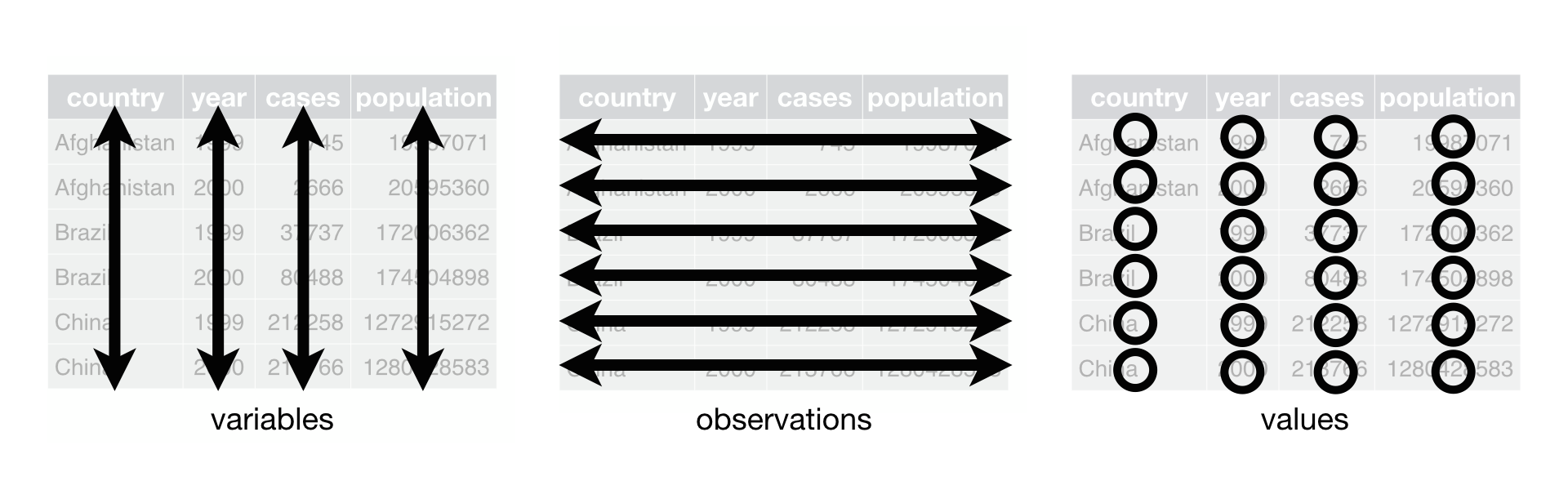#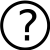Problem

• What is wrong?
``````plot(test_df[, 2:3],
main  = "Testplot",
lines(test_df[, 2:3])
points(test_df[1:3, 7:3],
pch = 17,
col = "redd",
cex = 3)
text(test_df[, 2:3],
labels = test_df[, 1],
pos    = 4
cex    = 1)``````

#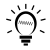Answer

• lines can not be a parameter of plot
• index 7:3 is not defined
• color “redd”
• comma after the 4
``````plot(test_df[, 2:3],
main  = "Testplot",
lines(test_df[, 2:3])
points(test_df[1:3, 7:3],
pch = 17,
col = "redd",
cex = 3)
text(test_df[, 2:3],
labels = test_df[, 1],
pos    = 4
cex    = 1)``````
``````plot(test_df[, 2:3],
main  = "Testplot")
lines(test_df[, 2:3])
points(test_df[1:3, 2:3],
pch = 17,
col = "red",
cex = 3)
text(test_df[, 2:3],
labels = test_df[, 1],
pos    = 4,
cex    = 1)``````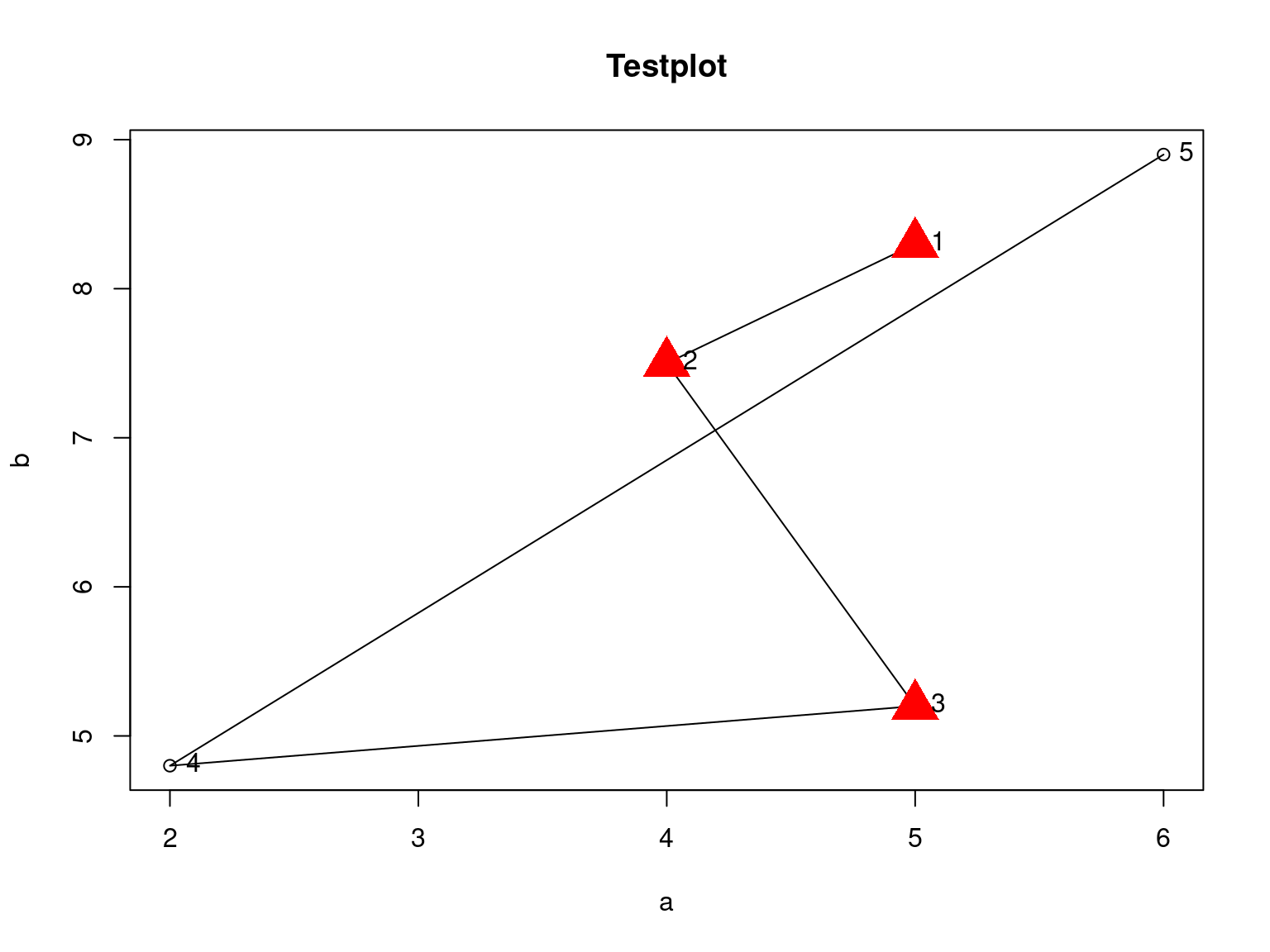#What is control flow?

# Control flow

• Loops repeat code
• `for()` certain number of repetitions
• `while()` as long as a condition is met
• `repeat()` until we break the loop
• Conditions run code if a condition is met
• `if()` run some code or not
• `if() else()` run some code or another code
• simple examples (both would be solved differently in practice)
• calculate the mean artefact size for all sites
• calculate the mean size for complete artefacts only

# Control flow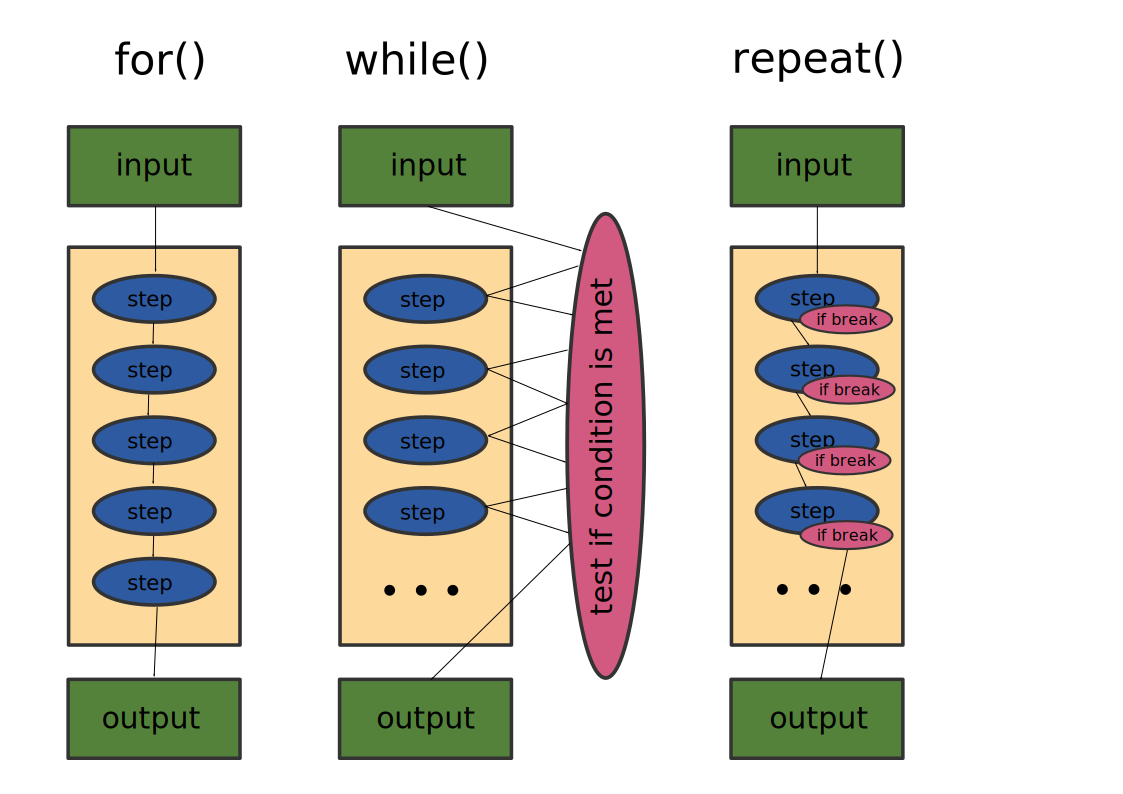# For

For loops repeat code a certain times and use an internal iteration variable.

``````for (i in 1:5){
print(paste("Loop no.: ", i))
}``````
``````##  "Loop no.:  1"
##  "Loop no.:  2"
##  "Loop no.:  3"
##  "Loop no.:  4"
##  "Loop no.:  5"``````

# While

While loops repeat code until a certain condition is met. Iteration variables, if required have to be installed manually.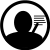Analyze Code!

``````i <- 1
while(i < 5){
print(paste("Loop no.: ", i))
i <- i + 1
}``````
``````##  "Loop no.:  1"
##  "Loop no.:  2"
##  "Loop no.:  3"
##  "Loop no.:  4"``````

# While

Iteration variable and the variable for the condition need not to be the same.

``````i <- 1
a <- 0
while(a < 5000){
a <- 2^i
print(paste("Loop no.: ", i))
i <- i + 1
}``````
``````##  "Loop no.:  1"
##  "Loop no.:  2"
##  "Loop no.:  3"
##  "Loop no.:  4"
##  "Loop no.:  5"
##  "Loop no.:  6"
##  "Loop no.:  7"
##  "Loop no.:  8"
##  "Loop no.:  9"
##  "Loop no.:  10"
##  "Loop no.:  11"
##  "Loop no.:  12"
##  "Loop no.:  13"``````

# If

If allows for conditional code. The condition contains a logical value and can make use of logical operators: `==, !=, <, <=, >, >=`

The terms can be combined with and `&` and or `|`.

``if(3 == 4){print("abc")}``

# Repeat

Repeat repeats code until we break the loop with `break`.

``````i <- 1
repeat{print(paste("Loop no.: ", i))
i <- i + 1
if(i < 5){next}
print(paste("Value: ", 2^i))
if(i > 10){break}
}``````
``````##  "Loop no.:  1"
##  "Loop no.:  2"
##  "Loop no.:  3"
##  "Loop no.:  4"
##  "Value:  32"
##  "Loop no.:  5"
##  "Value:  64"
##  "Loop no.:  6"
##  "Value:  128"
##  "Loop no.:  7"
##  "Value:  256"
##  "Loop no.:  8"
##  "Value:  512"
##  "Loop no.:  9"
##  "Value:  1024"
##  "Loop no.:  10"
##  "Value:  2048"``````

# If Else

If Else tests for a condition. If the condition is True Code 1 is terminated if False execute Code 2.

``````if(3 == 4){print("abc")
}else{print("deff")}``````
``##  "deff"``

# Conditions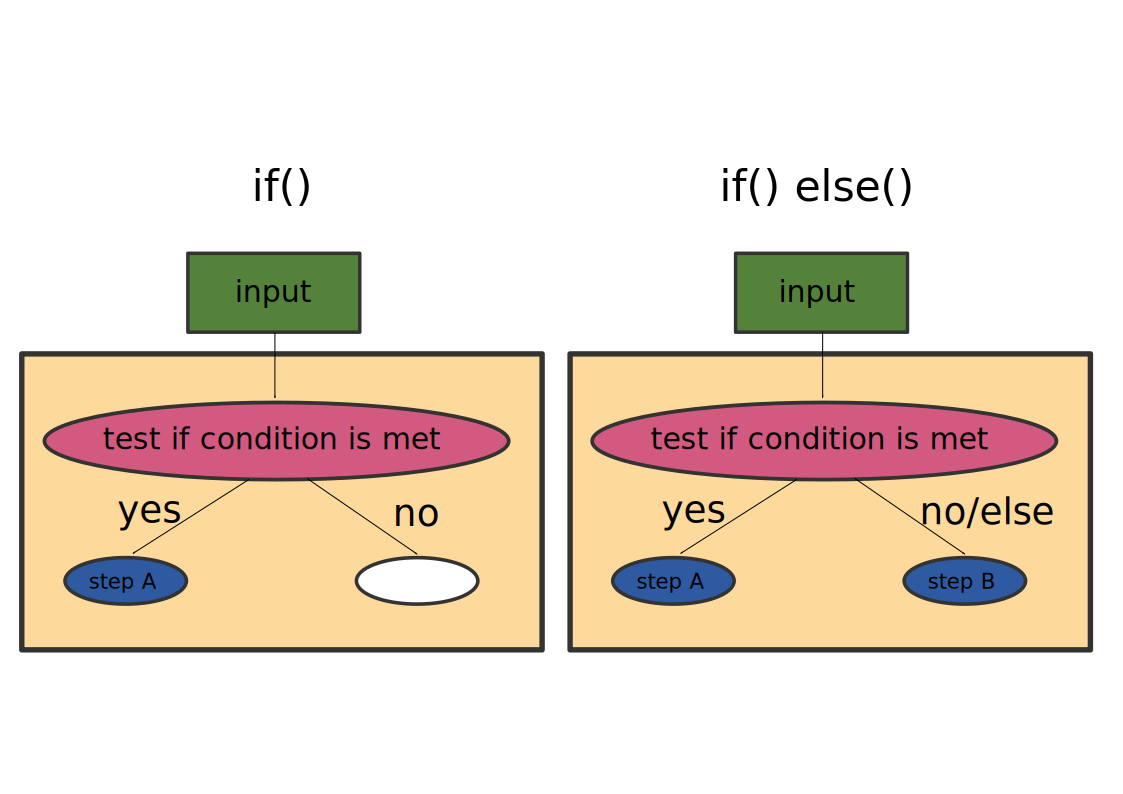#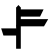• Loops are supposed to be slower and less elegant as vector based methods. In practice it is only relevant if they work and are fast enough for your purpose.

Functions are container, shortcuts or names for pieces of code. Variables can be passed on to the functions as parameters.analyze Code!

``````add <- function(a, b){
c <- a + b
return(c)
}``````
``add(3, 5)``
``##  8``

# Vector based methods: using vectors

``````x <- c(2, 4, 1, 5)
sqrt(x)``````
``##  1.414214 2.000000 1.000000 2.236068``
``mean(x)``
``##  3``
• `sqrt()` expects a number as a parameter or operates on a vector
• `mean()` expects a vector as parameter

# Vector based methods: `apply` and co.

`apply` is a function that runs other functions for every column or row of a matrix or dataframe. `apply` is usually faster than a loop.analyze Code!

``````df <- data.frame(a = c(1, 2, 3, 4, 5),
b = c(5, 4, 3, 2, 1),
c = c(3, 5, 3, 7, 5))
apply(df,
1,         # 1 for row; or 2 for column indexing
mean)``````
``##  3.000000 3.666667 3.000000 4.333333 3.666667``
``````apply(df,
2,
mean)``````
``````##   a   b   c
## 3.0 3.0 4.6``````

#Which approach do you prefer?

• Loop
• Apply

# Tidyverse

Tidyverse is a philosophy and a style of data sciences within the R ecosphere, initiated by Hadley Wickham (now at RStudio). Tidyverse includes R-packages partly as part of the meta-package `tidyverse`. The following slides are mainly based on Wickham/Grolemund (2017): http://r4ds.had.co.nz/.

• Data concept
• Packages for data handling
• Programming style

# Tidyverse

• Tidyverse-Packages:
• ggplot2
• tibble
• tidyr
• purrr
• dplyr
• stringr
• forcats
• magrittr
• modelr
• glue
• broom
``library("tidyverse")``

# Tidy data